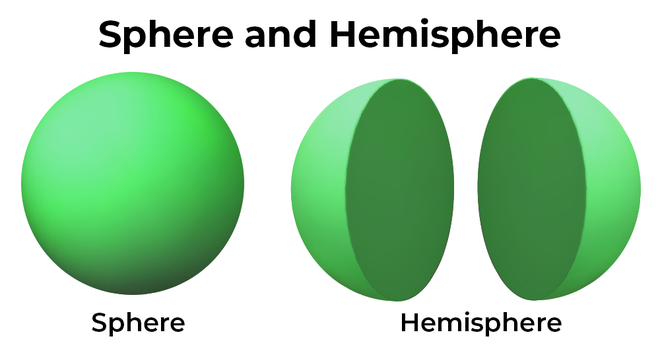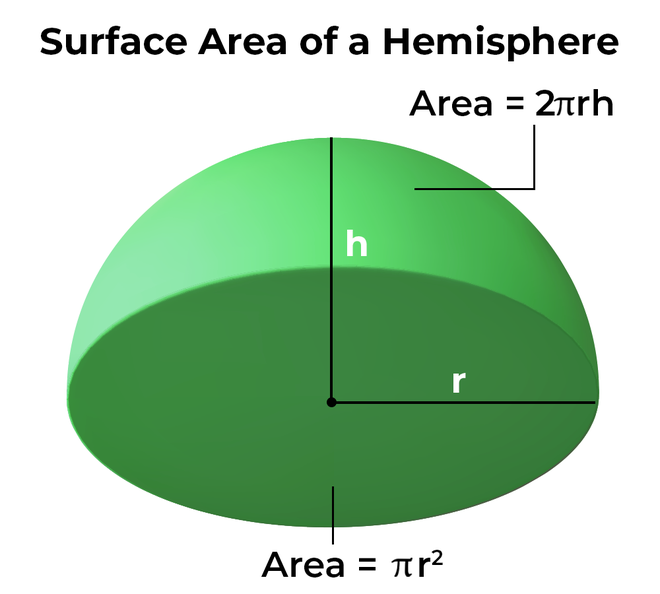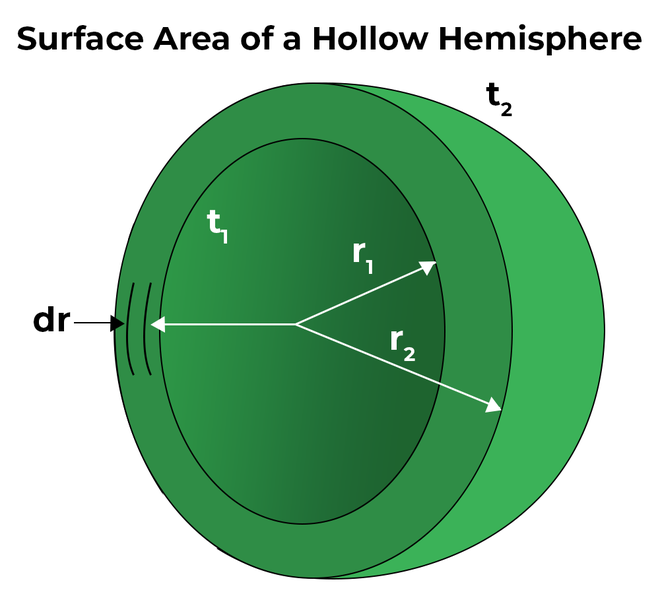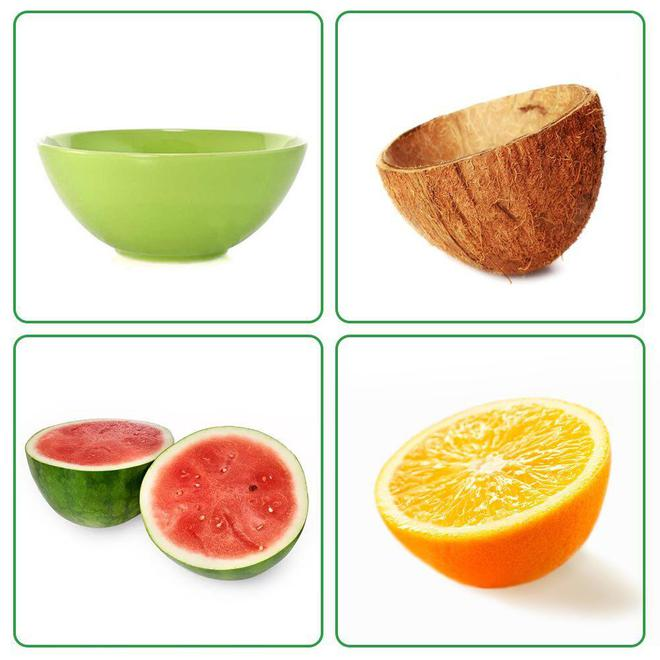Open in App
Not now

# Surface Area of a Hemisphere

• Last Updated : 06 Sep, 2022

The surface area of a hemisphere is the area covered by the faces of the hemisphere. The curved surface area and the base area sum up to make the total surface area of the hemisphere. The area of only the curved part is called the curved surface area of hemisphere. Before learning the formula for the surface area of hemisphere, let’s understand what is hemisphere followed by the formula and solved examples.

## What is the Surface Area of a Hemisphere?

The surface area of a hemisphere is also known as the total surface area of hemisphere. The total surface area of a hemisphere is the total area of all the faces (curved and base area). A hemisphere is formed when a plane divides a sphere into two equal parts. To put it another way, a hemisphere is an exact half of a sphere in geometry. It is made up of two parts: hemi, which means half, and sphere, which is a 3D mathematical shape. When a sphere is sliced at the precise center along its diameter, two equal hemispheres are generated. As a result, a hemisphere is a three-dimensional geometric object consisting of half of a sphere with one side flat and the other as a round bowl.## Surface Area of a Hemisphere Formula

The surface area of a hemisphere is the total surface area of a hemisphere. The surface area of hemisphere is defined by two types of hemispheres, the solid hemisphere, and the hollow hemisphere. The surface area can be found in two ways:

• The curved surface area of a hemisphere (CSA).
• The total surface area of a hemisphere (TSA).

### Curved Surface Area of Hemisphere Formula

The curved surface area of hemisphere formula is defined as the area covered by its curved surface. It is equal to half of the total surface area of a sphere. The formula for the curved surface area of hemisphere equals two times the product of pi and the square of the radius of the hemisphere.

Curved surface area of a hemisphere = 1/2 (Curved surface area of a sphere)

CSA = 1/2 (4πr2)

CSA = 2πr2

Therefore, the formula for curved surface area of hemisphere formula becomes,

CSA = 2πr2

Where,

CSA is the curved surface area,

π is a constant with the value of 3.14,

r is the radius of hemisphere.

### Base Area

The base of the hemisphere is in a circular shape, and therefore, the formula for the base area of the hemisphere is equal to the area of a circle.

Base Area = πr2

### Total Surface Area of a Hemisphere Formula

The total surface area of a hemisphere is defined as the total space covered by the surface of the hemisphere. The total surface area is given by the sum of its curved surface area and base area. The formula for total surface area equals three times the product of the pi (π) and the square of the radius of the hemisphere.Total surface area of a hemisphere = Curved surface area + Base area

TSA = 2πr2 + πr2

TSA = 3πr2

Therefore, the formula for the total surface area of a hemisphere becomes,

TSA = 3πr2

Where,

TSA is the total surface area,

π is a constant with the value of 3.14,

r is the radius of hemisphere.

### Surface Area of a Hollow Hemisphere Formula

The surface area of a hollow hemisphere formula should be first explained in order to understand the formula well. A hollow hemisphere has two diameters as the hollow inner hemisphere creates another smaller diameter. If noted carefully, it can be seen that the surface area of a hollow hemisphere consists of the curved surface area of the bigger hemisphere, the curved surface area of the smaller hemisphere, and the area of the remaining base. Let’s break down the areas in order to obtain the surface area of a hollow hemisphere,

• The curved surface area of the outer hemisphere = 2πR2
• The curved surface area of the inner hemisphere = 2πr2
• The base area of the hollow hemisphere = π(R2 – r2)

Therefore, the total surface area of the hemisphere = 2πR2 + 2πr2 + π(R2 – r2)

TSA = 2πR2 + 2πr2 + πR2 – πr2

TSA = 3πR2 + πr2## How to Find the Surface Area of a Hemisphere?

The surface area of a hemisphere can be found by following easy steps based on what type of hemisphere is given. If a solid hemisphere is given, the formula of total surface area and curved surface area can be used based on the requirement, and if a hollow hemisphere is given, the formula for a hollow hemisphere must be used. Following are the steps that can be followed to obtain the surface areas based on the requirement.

### Curved Surface Area of a Hemisphere

The formula for the curved surface area of a hemisphere when the given radius is “r” is 2πr2. Below are the steps provided to find the curved surface area of a hemisphere:

• Note down the radius of the hemisphere.
• Put the “r” value in the formula for the curved surface area of sphere, that is, CSA = 2πr2.
• Present the final answer in square units.

Example: Calculate the curved surface area of a hemisphere radius of 5 m. (Use π = 3.14).

Solution:

We have,

r = 5

Using the formula we get,

CSA = 2πr2

= 3 (3.14) (5)2

= 235.5 sq. m

### Total Surface Area of a Hemisphere

The formula for the total surface area of a hemisphere when the given radius is “r” is 3πr2. Below are the steps provided to find the curved surface area of a hemisphere:

• Note down the radius of the hemisphere.
• Put the “r” value in the formula for the total surface area of sphere, that is, TSA = 3πr2.
• Present the final answer in square units.

Example: Calculate the total surface area of a hemisphere diameter of 16cm.

Solution:

We have,

d = 16cm

r = 8cm

Using the formula we get,

TSA = 3πr2

= 3 (3.14) (8)2

= 602.88 sq. cm.

### Surface Area of a Hollow Hemisphere

The formula for the surface area of a hollow hemisphere when the given radius is “r” is 3πR2 + πr2. Below are the steps provided to find the surface area of a hollow hemisphere:

• Note down the radius of the hemisphere.
• Put the “r” value in the formula for the total surface area of a sphere, that is, TSA = 3πR2 + πr2.
• Present the final answer in square units.

### Real-Life Examples of Hemisphere

The examples of hemispheres can be seen in everyday life. For instance, the bowl that we use to eat food from is nothing but a hollow hemisphere. The half-cut shell of the coconut is an example of a hollow hemisphere. When we cut round-shaped fruits like oranges, tamarind, watermelon, etc., the shape of the fruit becomes a solid hemispherical shape. Below shown images are real-life examples of hemispheres.## Solved Example on Surface Area of Hemisphere

Example 1: Calculate the total surface area of a hemisphere radius of 4 m.

Solution:

We have,

r = 4

Using the formula we get,

TSA = 3πr2

= 3 (3.14) (4)2

= 150.72 sq. m

Example 2: Calculate the radius of a hemisphere if its total surface area is 200 sq. m.

Solution:

We have,

A = 200

Using the formula we get,

A = 3πr2

=> r2 = A/3π

=> r2 = 200/3 (3.14)

=> r = 4.60 m

Example 3: Calculate the radius of a hemisphere if its total surface area is 350 sq. m.

Solution:

We have,

A = 200

Using the formula we get,

A = 3πr2

=> r2 = A/3π

=> r2 = 350/3 (3.14)

=> r = 6.09 m

Example 4: Calculate the curved surface area of a hemisphere radius of 4 m.

Solution:

We have,

r = 4

Using the formula we get,

CSA = 2πr2

= 3 (3.14) (4)2

= 150.72 sq. m

Example 5: Calculate the radius of a hemisphere if its curved surface area is 790 sq. m.

Solution:

We have,

A = 790

Using the formula we get,

A = 2πr2

=> r2 = A/2π

=> r2 = 350/2 (3.14)

=> r = 7.46 m

## FAQs on Surface Area of a Hemisphere

Question 1: What is the lateral surface area of a hemisphere?

The lateral surface area of hemisphere is another name for the curved surface area of hemisphere and the formula for curved surface area of a hemisphere is 2πr2.

Question 2: How to find the base area of a hemisphere?

The base area of hemisphere has circular shape and therefore, the area of circle will be used to find the base area of hemisphere. Hence, base area becomes πr2.

Question 3: How to find the surface area of a hemisphere?

The formula for total surface area of a hemisphere when the given radius is “r” is 3πr2. Below are the steps provided to find curved surface area of a hemisphere:

• Note down the radius of the hemisphere.
• Put the “r” value in the formula for total surface area of sphere, that is, TSA = 3πr2.
• Present the final answer in square units.

Question 4: Explain the difference between the curved surface area of hemisphere and the surface area of a hemisphere.

The curved surface area of hemisphere is only the area covered by the curved surface and the formula for curved surface area of hemisphere is 2πr2. While the surface area of a hemisphere is the sum of the curved surface area and the base area of hemisphere, therefore, the formula for TSA becomes 3πr2.

Question 5: What is the surface area of a hemispherical shell?